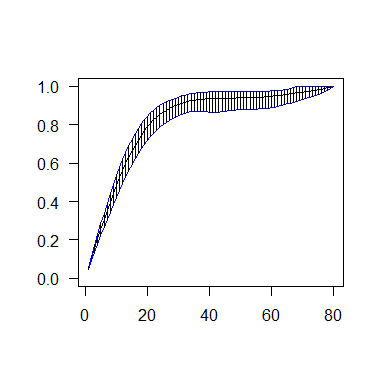Confidence intervals with current status data

2017-10-12

In the current status model, a positive variable of interest $$X$$ with distribution function $$F_0$$ is not observed directly. A censoring variable $$T \sim G$$ is observed instead together with the indicator $$\Delta=(X \le T)$$. curstatCI provides functions to estimate the distribution function $$F_0$$ and to construct pointswise confidence intervals around $$F_0(t)$$ based on an observed sample $$(T_1, \Delta_1),\ldots, (T_n, \Delta_n)$$ of size $$n$$ from the observable random vector $$(T, \Delta)$$. The methods used in this package are described in Groeneboom and Hendrickx (2017). More details on the current status model can be found in Groeneboom and Jongbloed (2014).

To illustrate the usage of the functions provided in the curstatCI package, we consider a sample $$(X_1,\ldots,X_n)$$ of size $$n=1000$$ from the truncated exponential distribution on $$[0,2]$$ with distribution function $$F_0(t)=(1-\exp(-t))/(1-\exp(-2)$$ if $$t \in [0,2]$$. The observation points $$(T_1,\ldots,T_n)$$ are sampled from a uniform distribution on $$[0,2]$$. This data setting is also considered in the simulation section of Groeneboom and Hendrickx (2017). The R-code to generate the observable random vector $$(t_1,\delta_1), \ldots, (t_n, \delta_n)$$ is given below.

set.seed(100)

n<-1000
t<-rep(NA, n)
delta<-rep(NA, n)

for(i in (1:n) ){
x<-runif(1)
y<--log(1-(1-exp(-2))*x)
t[i]<-2*runif(1);
if(y<=t[i]){ delta[i]<-1}
else{delta[i]<-0}}

The nonparametric maximum likelihood estimator (MLE)

The MLE $$F_n$$ of $$F_0$$ is defined as the maximizer of the log likelihood given by (up to a constant not depending on $$F$$) $\sum_{i=1}^n \Delta_i\log F(T_i) + (1-\Delta_i)\log(1-F(T_i)),$ over all possible distribution functions $$F$$. As can be seen from its structure, the log likelihood only depends on the value that $$F$$ takes at the observed time points $$T_i$$. The values in between are irrelevant as long as $$F$$ is increasing. The MLE $$F_n$$ is a step function which can be characterized by the left derivative of the greatest convex minorant of the cumulative sumdiagran consisting of the points $$P_0=(0,0)$$ and $P_i=\left(\sum_{j=1}^i w_j,\sum_{j=1}^i f_{1j}\right),\,i=1,\dots,m,$

where the $$w_j$$ are weights, given by the number of observations at point $$T_{(j)}$$, assuming that $$T_{(1)}<\dots<T_{(m)}$$ ($$m$$ being the number of different observations in the sample) are the order statistics of the sample $$(T_1,\Delta_1),\dots,(T_n,\Delta_n)$$ and where $$f_{1j}$$ is the number of $$\Delta_k$$ equal to one at the $$j$$th order statistic of the sample. When no ties are present in the data, $$w_j=1, m=n$$ and $$f_{1j}=\Delta_{(j)}$$, where $$\Delta_{(j)}$$ corresponds to $$T_{(j)}$$.

The function ComputeMLE in the package curstatCI computes the values of the MLE at the distinct jump points of the stepwise MLE. The current status data needs to be formatted as follows. The first column contains the observations $$t_{(1)}<\dots<t_{(m)}$$ in ascending order. The second and third columns contain the variables $$f_{1j}$$ and $$w_j$$ corresponding to $$t_{(j)}$$.

A<-cbind(t[order(t)], delta[order(t)], rep(1,n))
##              [,1] [,2] [,3]
## [1,] 0.0007901406    0    1
## [2,] 0.0064286250    0    1
## [3,] 0.0075551583    0    1
## [4,] 0.0121238651    0    1
## [5,] 0.0150197768    0    1
## [6,] 0.0179643347    0    1

The function ComputeMLE returns the jump points and corresponding values of the MLE.

library(Rcpp)
library(curstatCI)

mle<-ComputeMLE(data=A)
mle
##             x        mle
## 1  0.00000000 0.00000000
## 2  0.04461921 0.05405405
## 3  0.12463964 0.15000000
## 4  0.18832966 0.18181818
## 5  0.21623244 0.21428571
## 6  0.24208302 0.25000000
## 7  0.25054101 0.30555556
## 8  0.32536475 0.38461538
## 9  0.34771374 0.38888889
## 10 0.39735814 0.45454545
## 11 0.55754520 0.50000000
## 12 0.63327266 0.51515152
## 13 0.70567068 0.54838710
## 14 0.74357757 0.61363636
## 15 0.82979603 0.66666667
## 16 0.83427289 0.69230769
## 17 0.90577147 0.69902913
## 18 1.13136189 0.75000000
## 19 1.14422337 0.81967213
## 20 1.38870142 0.85714286
## 21 1.40659256 0.90000000
## 22 1.48428796 0.92307692
## 23 1.55291452 0.97297297
## 24 1.77735579 0.98666667
## 25 1.91620524 1.00000000

The number of jump points in the simulated data example equals 24. The MLE is zero at all points smaller than the first jump point 0.04461921 and one at all points larger than or equal to the last jump point 1.91620524. A picture of the step function $$F_n$$ is given below.

plot(mle$x, mle$mle,type='s', ylim=c(0,1),xlim=c(0,2), main="",ylab="",xlab="",las=1)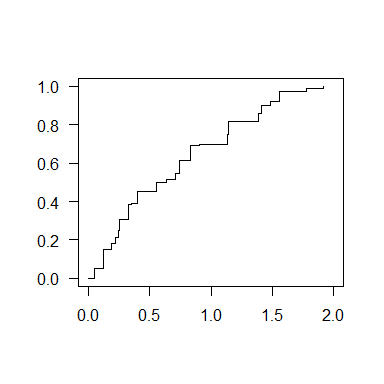The smoothed maximum likelihood estimator (SMLE)

Starting from the nonparametric MLE $$F_n$$, a smooth estimator $$\tilde{F}_{nh}$$ of the distribution function $$F_0$$ can be obtained by smoothing the MLE $$F_n$$ using a kernel function $$K$$ and bandwidth $$h>0$$. We use the triweight kernel defined by $K(t)=\frac{35}{32}\left(1-t^2\right)^31_{[-1,1]}(t).$ The SMLE is next defined by $\tilde F_{nh}(t)=\int\mathbb K\left(\frac{t-x}{h}\right)\,d F_n(x),$ where $\mathbb K(t)=\int_{-\infty}^t K(x)\,dx.$ The function ComputeSMLE computes the values of the SMLE in the points x based on a pre-specified bandwidth choice $$h$$. A user-specified bandwidth vector bw of size length(x) is used for each point in the vetor x. A data-driven bandwidth choice for each point in x is returned by the function ComputeBW which is described later.

For our simulated data set, a picture of the SMLE $$\tilde F_{nh}(t)$$ using a bandwidth $$h=2n^{-1/5}$$, evaluated in the points $$t=0.02,0.04,\ldots,1.98$$ together with the true distribution function $$F_0$$ is obtained as follows:

grid<-seq(0.02,1.98, 0.02)
bw<-rep(2*n^-0.2,length(grid))
smle<-ComputeSMLE(data=A, x=grid, bw=bw)
plot(grid, smle,type='l', ylim=c(0,1), main="",ylab="",xlab="",las=1)
lines(grid, (1-exp(-grid))/(1-exp(-2.0)), col=2, lty=2)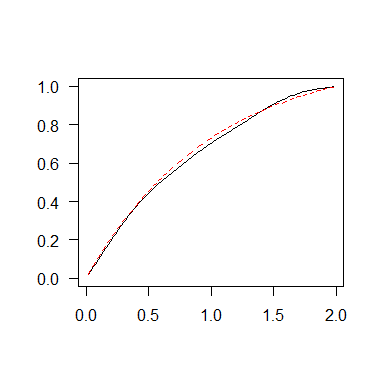Pointwise confidence intervals around the SMLE using a data-driven pointwise bandwidth vector

The nonparametric bootstrap procedure, which consists of resampling (with replacement) the $$(T_i,\Delta_i)$$ from the original sample is consistent for generating the limiting distribution of the SMLE $$\tilde F_{nh}(t)$$ under current status data (see Groeneboom and Hendrickx (2017)). As a consequence, valid pointwise confidence intervals for the distribution function $$F_0(t)$$ can be constructed using a bootstrap sample $$(T_1^*,\Delta_1^*),\ldots (T_n^*,\Delta_n^*)$$. The $$1-\alpha$$ bootstrap confidence intervals generated by the function ComputeConfIntervals are given by $\left[\tilde F_{nh}(t)-Q_{1-\alpha/2}^*(t)\sqrt{S_{nh}(t)}, \tilde F_{nh}(t)-Q_{\alpha/2}^*(t)\sqrt{S_{nh}(t)}\right],$ where $$Q_{\alpha}^*(t)$$ is the $$\alpha$$th quantile of $$B$$ values of $$W_{nh}^*(t)$$ defined by, $W_{nh}^*(t)=\left\{\tilde F_{nh}^*(t)-\tilde F_{nh}(t)\right\}/\sqrt{S_{nh}^*(t)},$ where $$\tilde F_{nh}^*(t)$$ is the SMLE in the bootstrap sample and $$S_{nh}(t)$$ is given by: $S_{nh}(t)=\frac1{(nh)^{2}}\sum_{i=1}^n K\left(\frac{t-T_i}h\right)^2\left(\Delta_i-F_n(T_i)\right)^2.$ $$S_{nh}^*(t)$$ is the bootstrap analogue of $$S_{nh}(t)$$ obtained by replacing $$(T_i,\Delta_i)$$ in the expression above by $$(T_i^*,\Delta_i^*)$$. The number of bootstrap samples $$B$$ used by the function ComputeSMLE equals $$B=1000$$.

The bandwidth for estimating the SMLE $$\tilde F_{nh}$$ at point $$t$$, obtained by minimizing the pointwise Mean Squared Error (MSE) using the subsampling principle in combination with undersmoothing is given by the function ComputeBW. To obtain an approximation of the optimal bandwidth minimizing the pointwise MSE, $$1000$$ bootstrap subsamples of size $$m=o(n)$$ are generated from the original sample using the subsampling principle and $$c_{t, opt}$$ is selected as the minimizer of $\sum_{b=1}^{1000}\left\{\tilde F_{m,cm^{-1/5}}^b(t) - \tilde F_{n,c_0n^{-1/5}}(t) \right\}^2,$ where $$\tilde F_{n,c_0n^{-1/5}}$$ is the SMLE in the original sample of size $$n$$ using an initial bandwidth $$c_0n^{-1/5}$$. The bandwidth used for estimating the SMLE is next given by $$h=c_{t,opt}n^{-1/4}$$.

When the bandwidth $$h$$ is small, it can happen that no time points are observed within the interval $$[t-h, t+h]$$. As a consequence the estimate of the variance $$S_{nh}(t)$$ is zero and the Studentized Confidence intervals are unsatisfactory. If this happens, the function ComputeConfIntervals returns the classical $$1-\alpha$$ confidence intervals given by: $\left[\tilde F_{nh}(t)-Z_{1-\alpha/2}^*(t), \tilde F_{nh}(t)-Z_{\alpha/2}^*(t)\right],$ where $$Z_{\alpha}^*(t)$$ is the $$\alpha$$th quantile of $$B$$ values of $$V_{nh}^*(t)$$ defined by, $V_{nh}^*(t)=\tilde F_{nh}^*(t)-\tilde F_{nh}(t).$

Besides the upper and lower bounds of the $$1-\alpha$$ bootstrap confidence intervals, the function ComputeConfIntervals also returns the output of the functions ComputeMLE and ComputeSMLE. The theory for the construction of pointwise confidence intervals is limited to points within the observation interval. It is not recomended to construct intervals in points smaller resp. larger than the smallst resp. largest observed time point.

The general approach for constructing the confidence intervals is the following: First decide upon the points where the confidence intervals need to be computed. If no particular interest in certain points is requested, it is useful to consider a grid of points within the interval starting from the smallest observed time point $$t_i$$ until the largest observed time point $$t_j$$. The function ComputeConfIntervals can also deal with positive values outside this interval but some numerical instability is to be expected and the results are no longer trustworthy.

c(min(t),max(t))
##  0.0007901406 1.9997072918
grid<-seq(0.01,1.99 ,by=0.01)

Next, select the bandwidth vector for estimating the SMLE $$\tilde F_{nh}(t)$$ for each point in the grid. If no pre-specified bandwidth value is preferred, a data-driven bandwidth vector can be obtained by the function ComputeBW.

bw<-ComputeBW(data=A, x=grid)
## The computations took    4.025   seconds
plot(grid, bw, main="",ylim=c(0.5,0.7),ylab="",xlab="",las=1)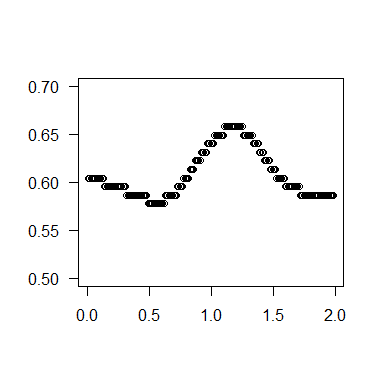The bandwidth vector obatined by the function ComputeBW is used as input bandwidth for the function ComputeConfIntervals.

out<-ComputeConfIntervals(data=A,x=grid,alpha=0.05, bw=bw)
## The program produces the Studentized nonparametric bootstrap confidence intervals for the cdf, using the SMLE.
##
## Number of unique observations:   1000
## Sample size n = 1000
## Number of Studentized Intervals = 199
## Number of Non-Studentized Intervals = 0
## The computations took    4.075   seconds

The function ComputeConfIntervals informs about the number of times the Studentized resp. classical bootstrap confidence intervals are calculated. The default method is the Studentized bootstrap confidence interval. In this simulated data example, the variance estimate for the Studentized confidence intervals is available for each point in the grid and out$Studentized equals grid. attributes(out) ##$names
##  "MLE"            "SMLE"           "CI"             "Studentized"
##  "NonStudentized"
out$NonStudentized ## numeric(0) A picture of the the SMLE together with the pointwise confidence intervals in the gridpoints and the true distribution function $$F_0$$ is given below: left<-out$CI[,1]
right<-out$CI[,2] plot(grid, out$SMLE,type='l', ylim=c(0,1), main="",ylab="",xlab="",las=1)
lines(grid, left, col=4)
lines(grid, right, col=4)
segments(grid,left, grid, right)
lines(grid, (1-exp(-grid))/(1-exp(-2.0)), col=2)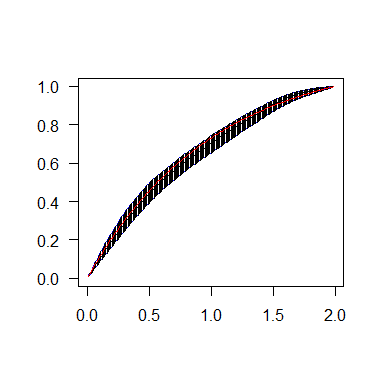Data applications

Hepatitis A data

Niels Keiding (1991) considered a cross-sectional study on the Hepatitis A virus from Bulgaria. In 1964 samples were collected from school children and blood donors on the presence or absence of Hepatitis A immunity. In total $$n=850$$ individuals ranging from 1 to 86 years old were tested for immunization. It is assumed that, once infected with Hepatitis A, lifelong immunity is achieved. To estimate the sero-prevalence for Hepatitis A in Bulgaria, 95% confidence intervals around the distribution function for the time to infection are computed using the ComputeConfIntervals function in the package curstatCI. Since only 22 out of the 850 individuals were older than 75 years, who, moreover, all had antibodies for Hepatitis A, it seems sensible to restrict the range to [1,75]. The resulting confidence intervals are obtained as follows:

data(hepatitisA)
##   t freq1 freq2
## 1 1     3    16
## 2 2     3    15
## 3 3     3    16
## 4 4     4    13
## 5 5     7    12
## 6 6     4    15
grid<-1:75

bw<-ComputeBW(data=hepatitisA,x=grid)
out<-ComputeConfIntervals(data=hepatitisA,x=grid,alpha=0.05, bw=bw)

The estimated prevalence of Hepatitis A at the age of 18 is 0.51, about half of the infections in Bulgaria happen during childhood.

out$SMLE ##  0.5109369 left<-out$CI[,1]
right<-out$CI[,2] plot(grid, out$SMLE,type='l', ylim=c(0,1), main="",ylab="",xlab="",las=1)
lines(grid, left, col=4)
lines(grid, right, col=4)
segments(grid,left, grid, right)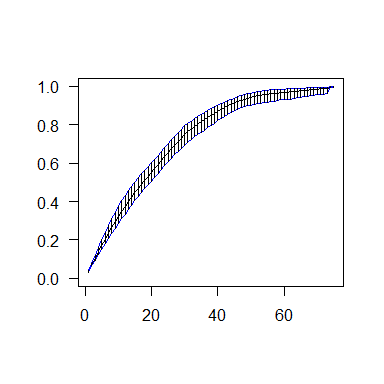The confidence interval around $$\tilde F_{nh}(1)$$ is computed using the classical confidence interval instead of the Studentized confidence interval.

out$NonStudentized ##  1 Rubella N. Keiding et al. (1996) considered a current status data set on the prevalence of rubella in 230 Austrian males with ages ranging from three months up to 80 years. Rubella is a highly contagious childhood disease spread by airborne and droplet transmission. The symptoms (such as rash, sore throat, mild fever and swollen glands) are less severe in children than in adults. Since the Austrian vaccination policy against rubella only vaccinated girls, the male individuals included in the data set represent an unvaccinated population and (lifelong) immunity could only be acquired if the individual got the disease. Pointwise confidence intervals are useful to investigate the time to immunization (i.e. the time to infection) against rubella. data(rubella) head(rubella) ## t freq1 freq2 ## 1 0.2740 0 1 ## 2 0.3781 0 1 ## 3 0.5288 0 1 ## 4 0.5342 0 1 ## 5 0.9452 1 1 ## 6 0.9479 0 1 summary(rubella$t)
##    Min. 1st Qu.  Median    Mean 3rd Qu.    Max.
##   0.274   8.868  25.595  28.970  44.888  80.118
grid<-1:80
bw<-ComputeBW(data=rubella,x=grid)
out<-ComputeConfIntervals(data=rubella,x=grid,alpha=0.05, bw=bw)

The SMLE increases steeply in the ages before adulthood which is in line with the fact that rubella is considered as a childhood disease.

left<-out$CI[,1] right<-out$CI[,2]

plot(grid, out\$SMLE,type='l', ylim=c(0,1), main="",ylab="",xlab="",las=1)
lines(grid, left, col=4)
lines(grid, right, col=4)
segments(grid,left, grid, right)